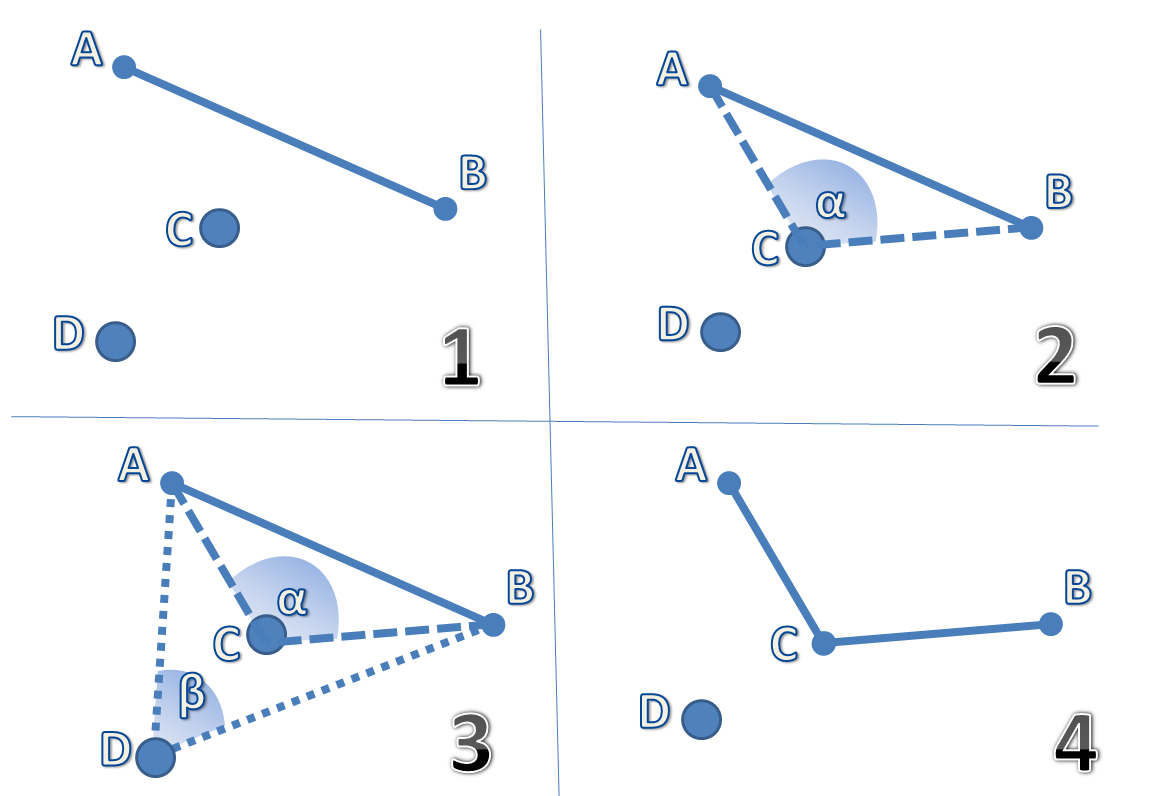Unity 2018.3.8f1OtherUpdated 228 days agoCreated on September 11th, 2016
Go to source

# Concave hull generator

Unite all dots under the smallest possible area. It comes with a simple demo for the Unity game engine using just gizmos.

## How it works

The program has only two easy steps:

• Set a convex hull from a set of points (In the program they are the `Node` objects). It is very important that they all have an unique `id` value. The hull should be a list of `Line` objects (each line is defined by two `Node` objects). If you already have a set of lines you can skip the step (They don’t need to form a convex hull)
• Set a concave hull from a list of `Line` objects.

You only need to set two constants before running the program:

• concavity : This sets how sharp you want the concave angles to be. It goes from `-1` (not concave at all. in fact, the hull will be left convex) up to `+1` (very sharp angles can occur. Setting concavity to `+1` might result in 0º angles!) `concavity` is defined as the cosine of the concave angles.
• scaleFactor : This sets how big is the area where concavities are going to be searched. The bigger, the more sharp the angles can be. Setting it to a very high value might affect the performance of the program. This value should be relative to how close to each other the points to be connected are.

For more info you can check the `Init.cs` file and follow the demo 😃## Algorithm

Inspired by Implementation of a fast and efficient concave hull algorith, the concave hull is reached through the iteration of four basic steps:

1. We find the points close to the longest edge of a hull. The distance is directly related to the value of `scaleFactor`
2. We measure the angle (in reality the cosine of this angle) of every point related to this segment.
3. We will select the point that gives us the widest angle/smallest cosine. If the value of the cosine is superior to the value of `concavity` we will discard this point.
4. The newly found point will be used to divide the segment into two new ones. The process will be repeated until no more divisions are made.Show all projects by Liagson